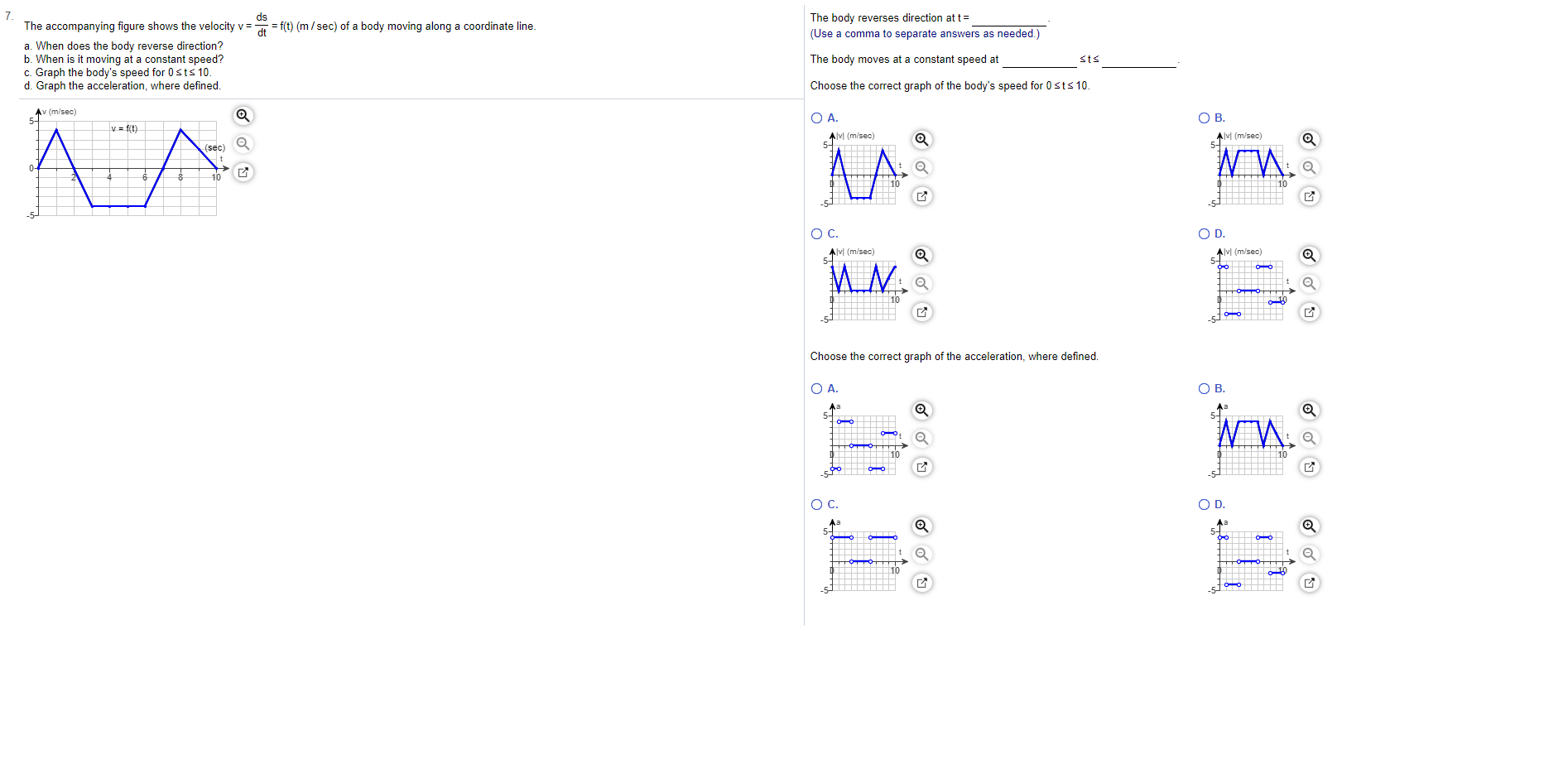# 7The accompanying figure shows the velocity vThe body reverses direction at t(Use a comma to separate answers as needed.)dsf(t) (m/sec) of a body moving along a coordinate line.dta. When does the body reverse direction?b. When is it moving at a constant speed?c. Graph the body's speed for 0 sts 10.d. Graph the acceleration, where defined.The body moves at a constant speed atstsChoose the correct graph of the body's speed for 0 sts10.Av (m/sec)O A.ОВ.Av (m/sec)5-Avi (m/sec)(sec)10гАO D.Ос.Av (m/sec)AvI (m/sec)MN10Choose the correct graph of the acceleration, where definedO B.O A.O D.Ос.г

Question
3 views

Can you help me step by step?help_outlineImage Transcriptionclose7 The accompanying figure shows the velocity v The body reverses direction at t (Use a comma to separate answers as needed.) ds f(t) (m/sec) of a body moving along a coordinate line. dt a. When does the body reverse direction? b. When is it moving at a constant speed? c. Graph the body's speed for 0 sts 10. d. Graph the acceleration, where defined. The body moves at a constant speed at sts Choose the correct graph of the body's speed for 0 sts10. Av (m/sec) O A. ОВ. Av (m/sec) 5- Avi (m/sec) (sec) 10 гА O D. Ос. Av (m/sec) AvI (m/sec) MN 10 Choose the correct graph of the acceleration, where defined O B. O A. O D. Ос. г fullscreen
check_circle

Step 1

Note: Since we only answer up to 3 sub-parts, we’ll answer the first 3.

Please resubmit the question and specify the other subparts (up to 3) you’d like answered.

For a body moving along a coordinate line it is required to determine the following from the graph given below:

Part a) The time when the body reverse direction.

Part b) The time when the body is moving with a constant speed.

Part c) Speed-time graph for the body on the time interval 0 to 10 seconds.

Step 2

Part a)

When a body moving along a coordinate axis changes its direction the sign of the velocity changes.

Observe form the velocity-time graph given below that the velocity of the body changes from positive to negative at the point x = 2 and from negative to positive at the point x = 7.

Step 3

Part b)

When the body is moving with a constant speed the velocity-time graph of the body is a straight line parallel to the x-axis.

Observe that...

### Want to see the full answer?

See Solution

#### Want to see this answer and more?

Solutions are written by subject experts who are available 24/7. Questions are typically answered within 1 hour.*

See Solution
*Response times may vary by subject and question.
Tagged in

### Calculus